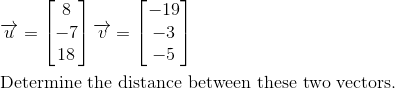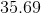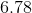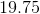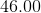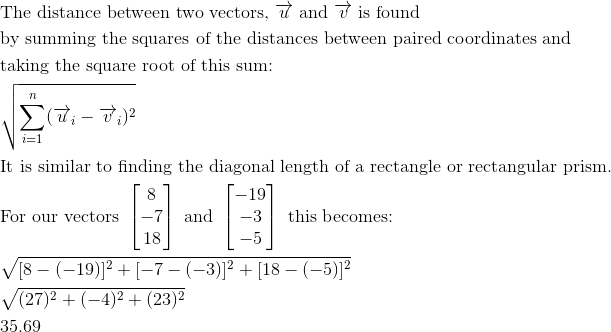Calculus 3 : Vectors and Vector Operations

Example Questions

Example Question #26 : Distance Between Vectors

Find the distance between the vectors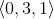and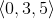Explanation:

To find the distance between two vectors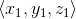and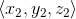, we use the formula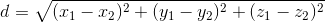Applying to the vectors from the problem statement, we get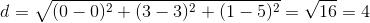Example Question #27 : Distance Between Vectors

Find the distance between the vectors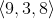and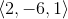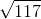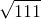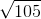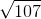Explanation:

To find the distance between two vectors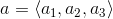and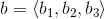, we use the formula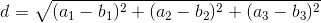Using the vectors from the problem statement, we get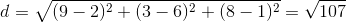Example Question #28 : Distance Between Vectors

Find the distance between the vectors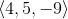and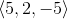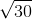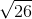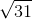Explanation:

To find the distance between two vectorsand, we use the formulaUsing the vectors from the problem statement, we get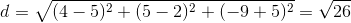Example Question #29 : Distance Between Vectors

Find the distance between the vectors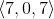and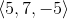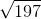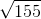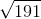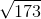Explanation:

To find the distance between two vectorsand, we use the formulaUsing the vectors from the problem statement, we get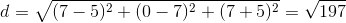Example Question #30 : Distance Between Vectors

Find the distance between the vectors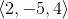and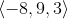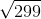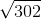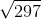Explanation:

To find the distance between two vectorsand, you use the formulaUsing the vectors from the problem statement, we get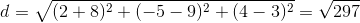Example Question #31 : Distance Between Vectors

Find the distance between the vectors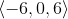and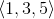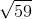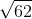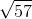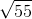Explanation:

To find the distance between two vectorsand, you use the formulaUsing the vectors from the problem statement, we get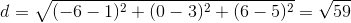Example Question #32 : Distance Between Vectors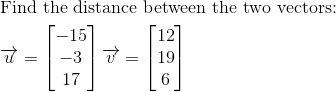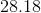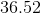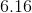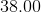Explanation: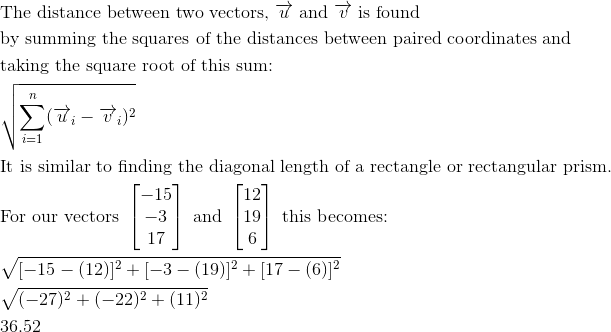Example Question #33 : Distance Between Vectors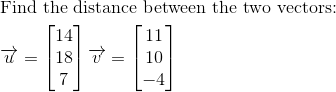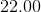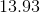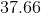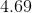Explanation: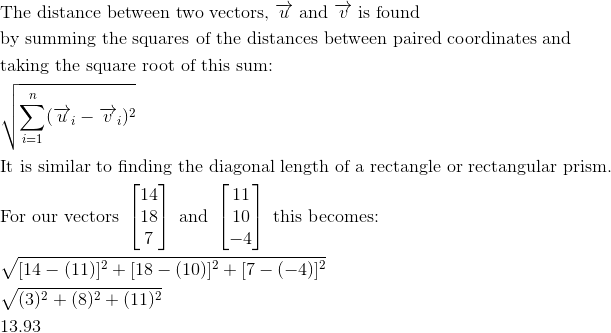Example Question #34 : Distance Between Vectors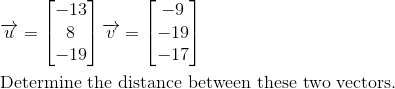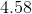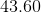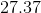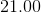Explanation: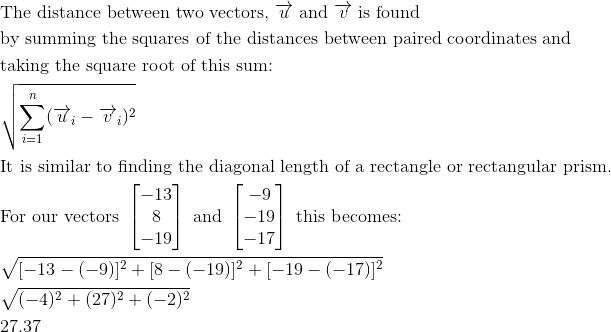Example Question #35 : Distance Between Vectors